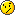### Welcome to Smart Home Forum by FIBARO

Dear Guest,

as you can notice parts of Smart Home Forum by FIBARO is not available for you. You have to register in order to view all content and post in our community. Don't worry! Registration is a simple free process that requires minimal information for you to sign up. Become a part of of Smart Home Forum by FIBARO by creating an account.

As a member you can:

•     Start new topics and reply to others
•     Get your own profile page and make new friends
•     Send personal messages
•     ... and learn a lot about our system!

Regards,

Smart Home Forum by FIBARO Team

• 0

# Can't change buttons now on KeyFob

## Question

Hi,

I can't change the buttons on the KeyFob now after the initial setupI tried to add a hidden scene manually (deleted the old button then added a new one) but it didn't work either.

I want to have the same options that I had from the beginning, where I could on the KeyFob device change the buttons, can anyone help me please?

## Recommended Posts

• 0

Why don't you use a lua scene for the key fob?

After:

Then

fibaro:debug('key')

You put as bellow:

---------------------------

if﻿(tonumber(fibaro:getValue(ID lamp, "value")) >= 1)
then
﻿ fibaro:call(ID lamp, "turnOff")
else
then
fibaro:c﻿all(ID lamp﻿, "turnOn")﻿

---------------------------

as below is the lua code.

--[[
%% properties
%% events
593 CentralSceneEvent
%% globals
--]]

local sa = fibaro:getSourceTrigger()["event"]["data"]
--fibaro:debug(json.encode(sa))

-- button square
if ((sa['keyId'] == 1) and (sa['keyAttribute'] ==  'Pressed'))
then
fibaro:debug('key1')
end

if ((sa['keyId'] == 1) and (sa['keyAttribute'] ==  'Pressed2'))
then
fibaro:debug('key1 double')
end

if ((sa['keyId'] == 1) and (sa['keyAttribute'] ==  'Pressed3'))
then
fibaro:debug('key1 triple')
end

if ((sa['keyId'] == 1) and (sa['keyAttribute'] ==  'HeldDown'))
then
fibaro:debug('key1 held')
end

if ((sa['keyId'] == 1) and (sa['keyAttribute'] ==  'Released'))
then
fibaro:debug('key1 release')
end

-- button circle
if ((sa['keyId'] == 2) and (sa['keyAttribute'] ==  'Pressed'))
then
fibaro:debug('key2')
end

if ((sa['keyId'] == 2) and (sa['keyAttribute'] ==  'Pressed2'))
then
fibaro:debug('key2 double')
end

if ((sa['keyId'] == 2) and (sa['keyAttribute'] ==  'Pressed3'))
then
fibaro:debug('key2 triple')
end

if ((sa['keyId'] == 2) and (sa['keyAttribute'] ==  'HeldDown'))
then
fibaro:debug('key2 held')
end

if ((sa['keyId'] == 2) and (sa['keyAttribute'] ==  'Released'))
then
fibaro:debug('key2 release')
end

-- button cross
if ((sa['keyId'] == 3) and (sa['keyAttribute'] ==  'Pressed'))
then
fibaro:debug('key3')
end

if ((sa['keyId'] == 3) and (sa['keyAttribute'] ==  'Pressed2'))
then
fibaro:debug('key3 double')
end

if ((sa['keyId'] == 3) and (sa['keyAttribute'] ==  'Pressed3'))
then
fibaro:debug('key3 triple')
end

if ((sa['keyId'] == 3) and (sa['keyAttribute'] ==  'HeldDown'))
then
fibaro:debug('key3 held')
end

if ((sa['keyId'] == 3) and (sa['keyAttribute'] ==  'Released'))
then
fibaro:debug('key3 release')
end

-- button triangle
if ((sa['keyId'] == 4) and (sa['keyAttribute'] ==  'Pressed'))
then
fibaro:debug('key4')
end

if ((sa['keyId'] == 4) and (sa['keyAttribute'] ==  'Pressed2'))
then
fibaro:debug('key4 double')
end

if ((sa['keyId'] == 4) and (sa['keyAttribute'] ==  'Pressed3'))
then
fibaro:debug('key4 triple')
end

if ((sa['keyId'] == 4) and (sa['keyAttribute'] ==  'HeldDown'))
then
fibaro:debug('key4 held')
end

if ((sa['keyId'] == 4) and (sa['keyAttribute'] ==  'Released'))
then
fibaro:debug('key4 release')
end

-- button minus
if ((sa['keyId'] == 5) and (sa['keyAttribute'] ==  'Pressed'))
then
fibaro:debug('key5')
end

if ((sa['keyId'] == 5) and (sa['keyAttribute'] ==  'Pressed2'))
then
fibaro:debug('key5 double')
end

if ((sa['keyId'] == 5) and (sa['keyAttribute'] ==  'Pressed3'))
then
fibaro:debug('key5 triple')
end

if ((sa['keyId'] == 5) and (sa['keyAttribute'] ==  'HeldDown'))
then
fibaro:debug('key5 held')
end

if ((sa['keyId'] == 5) and (sa['keyAttribute'] ==  'Released'))
then
fibaro:debug('key5 release')
end

-- button plus
if ((sa['keyId'] == 6) and (sa['keyAttribute'] ==  'Pressed'))
then
fibaro:debug('key6')
end

if ((sa['keyId'] == 6) and (sa['keyAttribute'] ==  'Pressed2'))
then
fibaro:debug('key6 double')
end

if ((sa['keyId'] == 6) and (sa['keyAttribute'] ==  'Pressed3'))
then
fibaro:debug('key6 triple')
end

if ((sa['keyId'] == 6) and (sa['keyAttribute'] ==  'HeldDown'))
then
fibaro:debug('key6 held')
end

if ((sa['keyId'] == 6) and (sa['keyAttribute'] ==  'Released'))
then
fibaro:debug('key6 release')
end

-- combinatie van verschillende knoppen
if (sa['keyId'] == 7)
then
fibaro:debug('key7')
fibaro:call(22, "turnOn")
end

if (sa['keyId'] == 8)
then
fibaro:debug('key8')
fibaro:call(22, "turnOff")
end

if (sa['keyId'] == 9)
then
fibaro:debug('key9')
end

if (sa['keyId'] == 10)
then
fibaro:debug('key10')
end

if (sa['keyId'] == 11)
then
fibaro:debug('key11')
end

if (sa['keyId'] == 12)
then
fibaro:debug('key12')
end

## Join the conversation

You can post now and register later. If you have an account, sign in now to post with your account.×   Pasted as rich text.   Paste as plain text instead

Only 75 emoji are allowed.

×   Your previous content has been restored.   Clear editor

×   You cannot paste images directly. Upload or insert images from URL.

×
×
• FIBARO.COM